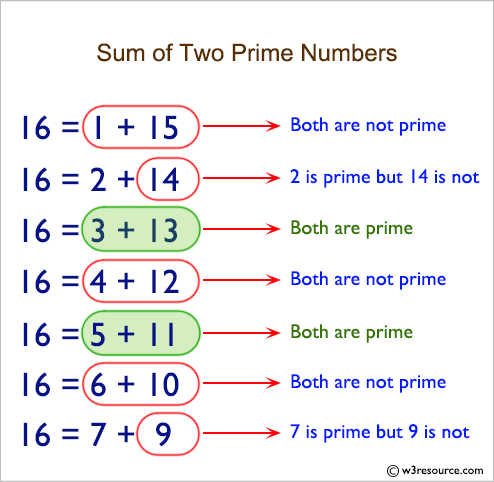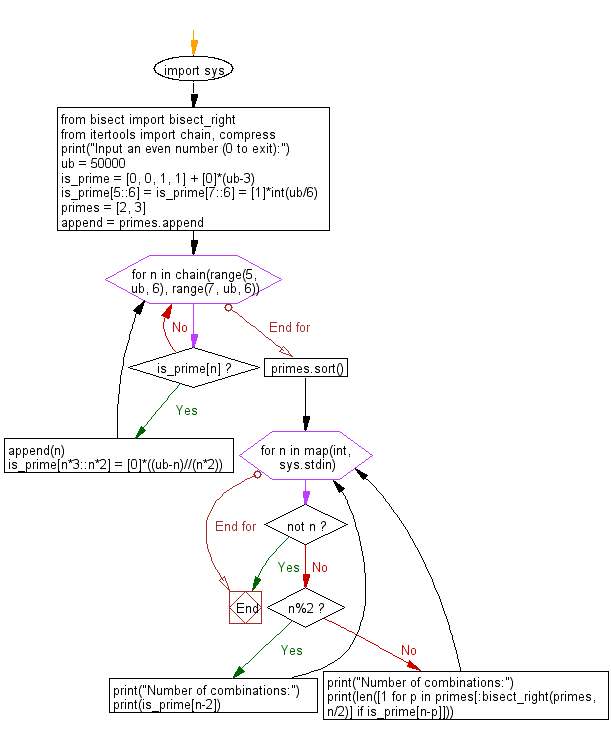﻿ Python: Accept an even number from the user and create a combinations that express the given number as a sum of two prime numbers - w3resource# Python: Accept an even number from the user and create a combinations that express the given number as a sum of two prime numbers

## Python Basic - 1: Exercise-53 with Solution

Write a Python program that accept an even number (>=4, Goldbach number) from the user and create a combinations that express the given number as a sum of two prime numbers. Print the number of combinations.

Goldbach number: A Goldbach number is a positive even integer that can be expressed as the sum of two odd primes. Since four is the only even number greater than two that requires the even prime 2 in order to be written as the sum of two primes, another form of the statement of Goldbach's conjecture is that all even integers greater than 4 are Goldbach numbers.
The expression of a given even number as a sum of two primes is called a Goldbach partition of that number. The following are examples of Goldbach partitions for some even numbers:
6 = 3 + 3
8 = 3 + 5
10 = 3 + 7 = 5 + 5
12 = 7 + 5
...
100 = 3 + 97 = 11 + 89 = 17 + 83 = 29 + 71 = 41 + 59 = 47 + 53

Pictorial Presentation:Sample Solution:

Python Code:

``````import sys
from bisect import bisect_right
from itertools import chain, compress
print("Input an even number (0 to exit):")
ub = 50000
is_prime = [0, 0, 1, 1] + *(ub-3)
is_prime[5::6] = is_prime[7::6] = *int(ub/6)
primes = [2, 3]
append = primes.append

for n in chain(range(5, ub, 6), range(7, ub, 6)):
if is_prime[n]:
append(n)
is_prime[n*3::n*2] = *((ub-n)//(n*2))
primes.sort()

for n in map(int, sys.stdin):
if not n:
break
if n%2:
print("Number of combinations:")
print(is_prime[n-2])
else:
print("Number of combinations:")
print(len([1 for p in primes[:bisect_right(primes, n/2)] if is_prime[n-p]]))
``````

Sample Output:

```Input an even number (0 to exit):
100
Number of combinations:
6
```

Flowchart:Python Code Editor:

Have another way to solve this solution? Contribute your code (and comments) through Disqus.

What is the difficulty level of this exercise?

Test your Programming skills with w3resource's quiz.

﻿

## Python: Tips of the Day

What is the difference between Python's list methods append and extend?

append: Appends object at the end.

```x = [1, 2, 3]
x.append([4, 5])
print (x)
```

Output:

```[1, 2, 3, [4, 5]]
```

extend: Extends list by appending elements from the iterable.

```x = [1, 2, 3]
x.extend([4, 5])
print (x)
```

Output:

```[1, 2, 3, 4, 5]
```

Ref: https://bit.ly/2AZ6ZFq